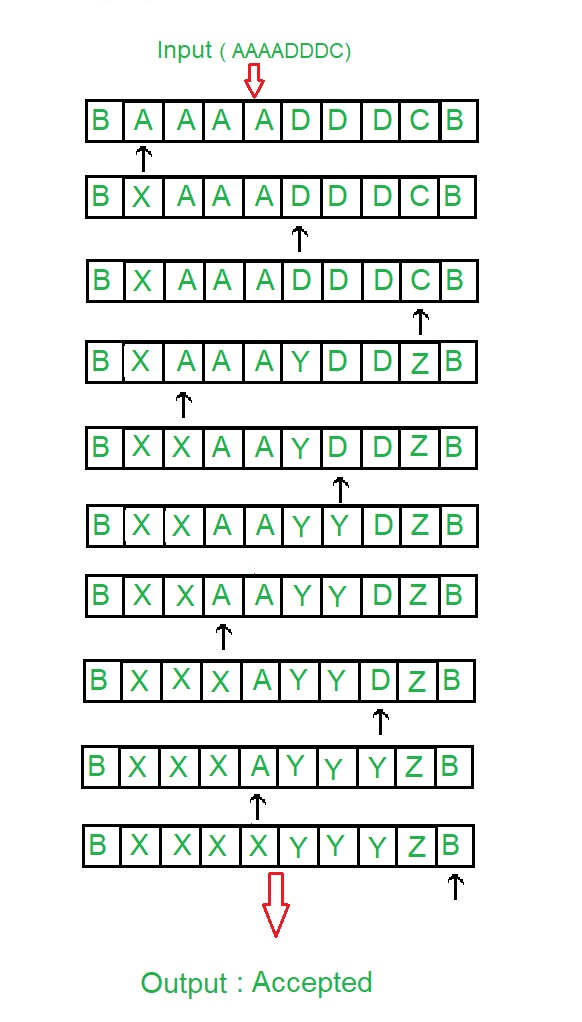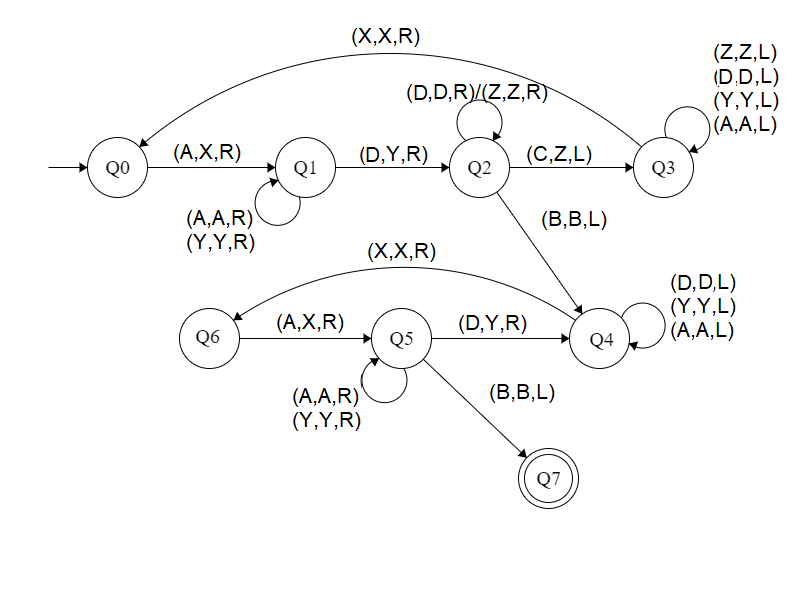Open In App

# Construct a Turing machine for L = {aibjck | i>j>k; k ≥ 1}

Prerequisite – Turing Machine

In given language L = {aibjck | i>j>k; k ≥ 1}, every string of ‘a’, ‘b’ and ‘c’ have certain number of a’s, then certain number of b’s and then certain number of c’s. The condition is that count of 3rd symbols should be atleast 1. ‘a’ and ‘b’ can have thereafter be as many but count of c is less than count of ‘b’ and count of ‘b’ is less than count of ‘a’. Assume that string is ending with ‘\$’.
Examples:

```Input: a a a b b c
Here a = 3, b = 2, c = 1
Output: ACCEPTED

Input: a a b b c c c
Here a = 2, b = 2, c = 3 but |a|>|b|>|c|
Output: NOT ACCEPTED ```

Tape Representation:Approach:

1. Comparing two elements by making A & D as a single element.
2. After that Comparing A & D.
3. If |C| is greater than |(A, D)|, then it is not accepted.
4. If |D| is greater than |A|, then it is not accepted.
5. Else it is accepted.

Steps:

• Step-1: Convert A into X and move right and goto step 2.

• Step-2: Keep ignoring A and Y and move towards right. Convert D into Y and move right and goto step-3.
• Step-3: Keep ignoring D and Z and move towards right.If C is found make it Z and move left to step 4.If B is found ignore it and move left and goto step-5.
• Step-4: Keep ignoring Z, A, Y and D and move towards left.If X is found ignore it and move right and goto step-1.
• Step-5: Keep ignoring D, Y and A and move towards left. Ignore X move right and goto step-6.
• Step-6: Convert A into X and move right and goto step-7.
• Step-7: Keep ignoring Y and A and move towards right.If B is found ignore it and move left and goto step-8.If D make it Y and move right and goto step-5.
• Step-8: Stop the Machine (String is accepted)

State transition diagram :Here, Q0 shows the initial state and Q1, Q2, Q3, Q4, Q5, Q6 shows the transition state and Q7 shows the final state. A, C, D are the variables used and R, L shows right and left.

Explanation:

• Using Q0, when A is found make it X and go to right and to state Q1.
• On the state Q1, ignore all A and Y and goto right.If D found make it Y and goto right into next state Q2.
• In Q2, ignore all D, Z and move right.If B found ignore it, move left and goto the state Q4, If C found make it Z move left and to Q3.
• In Q3 state, ignore all Z, D, Y, A and move left.If X found ignore it move right to Q0.
• In Q4, ignore all A, Y, D and move left.If X found ignore it move right to state Q6.
• In Q6 state, if A found make it X move right to state Q5
• In Q5, ignore all A, Y and move right.If D found make it Y and move right to state Q4.If B is found ignore it and move left to Q7
• If Q7 state is reached it will produced the result of acceptance of string.

Note: For comparison of |A|, |D|, |C|, the concept of Turing Machine as Comparator is used.